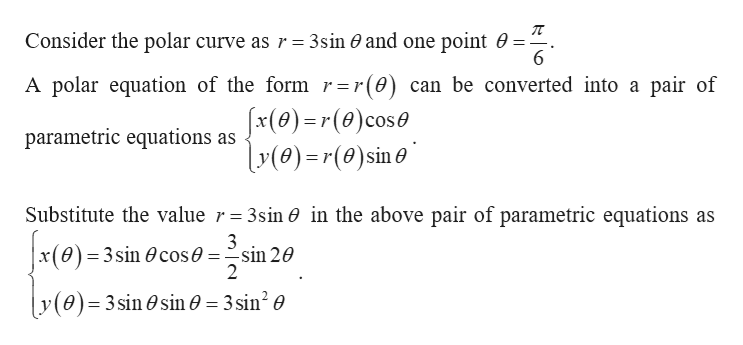# Find the slope of the tangent line to the given polar curve at the point specified by the value of θ.r = 3sin(θ)θ = π/6

Question
36 views

Find the slope of the tangent line to the given polar curve at the point specified by the value of θ.
r = 3sin(θ)
θ = π/6

check_circle

Step 1help_outlineImage TranscriptioncloseConsider the polar curve as r =3sin 0 and one point 0 = 6 A polar equation of the form r=r(0) can be converted into a pair of x(0)r(0) cos y0)=r(0)sin parametric equations as Substitute the value r = 3sin 0 in the above pair of parametric equations as 3 x(0) 3sin 0cos0 = sin 20 2 y(0) 3 sin 0sin0 = 3sin2 0 fullscreen

### Want to see the full answer?

See Solution

#### Want to see this answer and more?

Solutions are written by subject experts who are available 24/7. Questions are typically answered within 1 hour.*

See Solution
*Response times may vary by subject and question.
Tagged in

### Other